# 量子電腦系列：量子力學真的能做到瞬間移動嗎？

## 定義

$\left| \psi \right> = \alpha \left| 0 \right> + \beta \left| 1 \right>$

$$\alpha^2$$ 和 $$\beta^2$$ 分別表示量子比特測量後結果為 0 和 1 的可能性。所以透過這兩個變數可以包含單個量子比特的所有可能狀態。

## 量子閘

### X閘 (X Gate)

X gate 相當於邏輯閘的「非」門。下圖是 X gate 的真值表，每一行表示當這個閘門的輸入是左列的數值的話，輸出就是右列的值。例如把 $$\left| 0 \right>$$ 輸入給 X gate，輸出就會是 $$\left| 1 \right>$$ 。你也可以把它們想像成類似販賣機的機器，丟個零錢進去，換個飲料出來。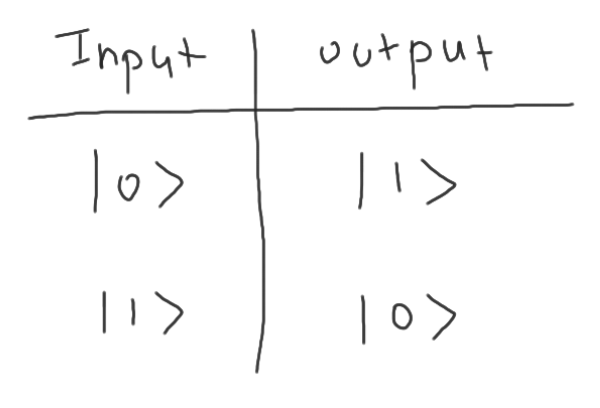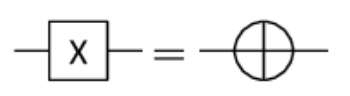X gate 有兩種表示法，右邊的形式比較常出現在等等會提到的 CNOT gate。

Hadamard gate 又稱 H gate。H gate 在量子演算法裡被大量地被運用，原因是它可以把一個在非疊加態的量子比特例如 $$\left| 0 \right>$$ 轉換成疊加態。 H gate 同時也是產生糾纏態 (Entanglement) 線路的必要零件。以下是它的真值表：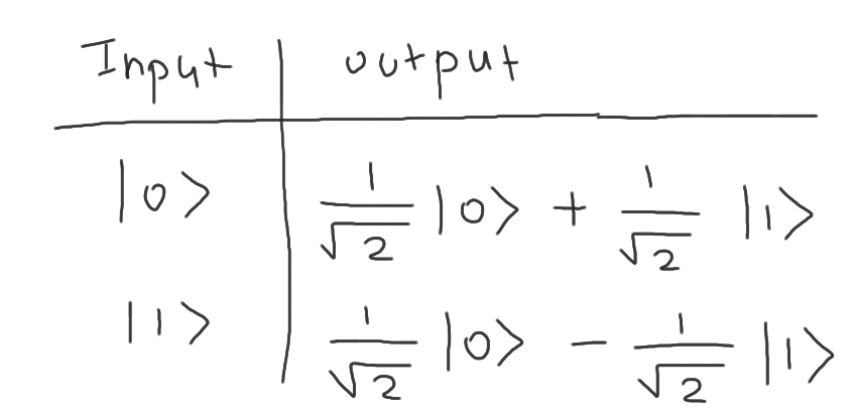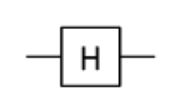### Z閘 (Z Gate)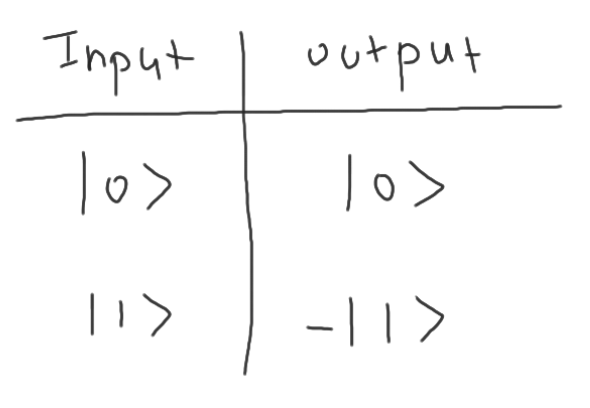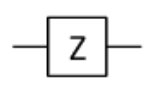### CNOT閘 (Controlled-Not Gate 或 CNOT Gate)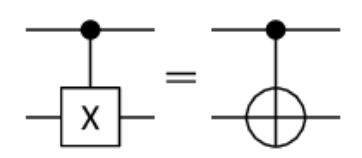CNOT gate 有兩個輸入，第一個輸入是圖中 X gate 的開關，第二個輸入則是會通過 X gate。這個 X gate 會根據第一個量子比特輸入的數值來決定要不要作用。只有當第一個輸入是 $$\left| 1 \right>$$ 時，X gate 才會起作用。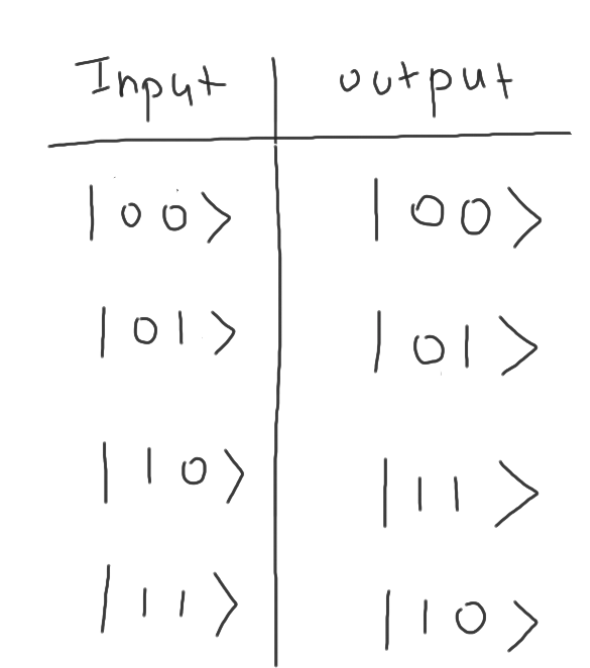### CZ閘 (Controlled-Z Gate 或 CZ Gate)

CZ 和 CNOT 類似，只是把 X gate 換成 Z gate。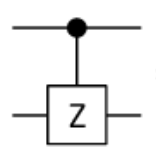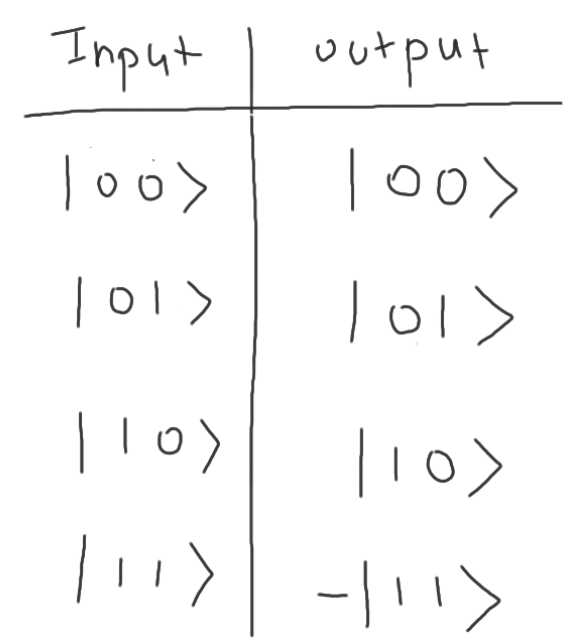## 測量 (Measurement)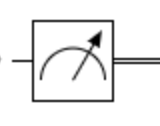## 量子傳送

### 量子傳送線路圖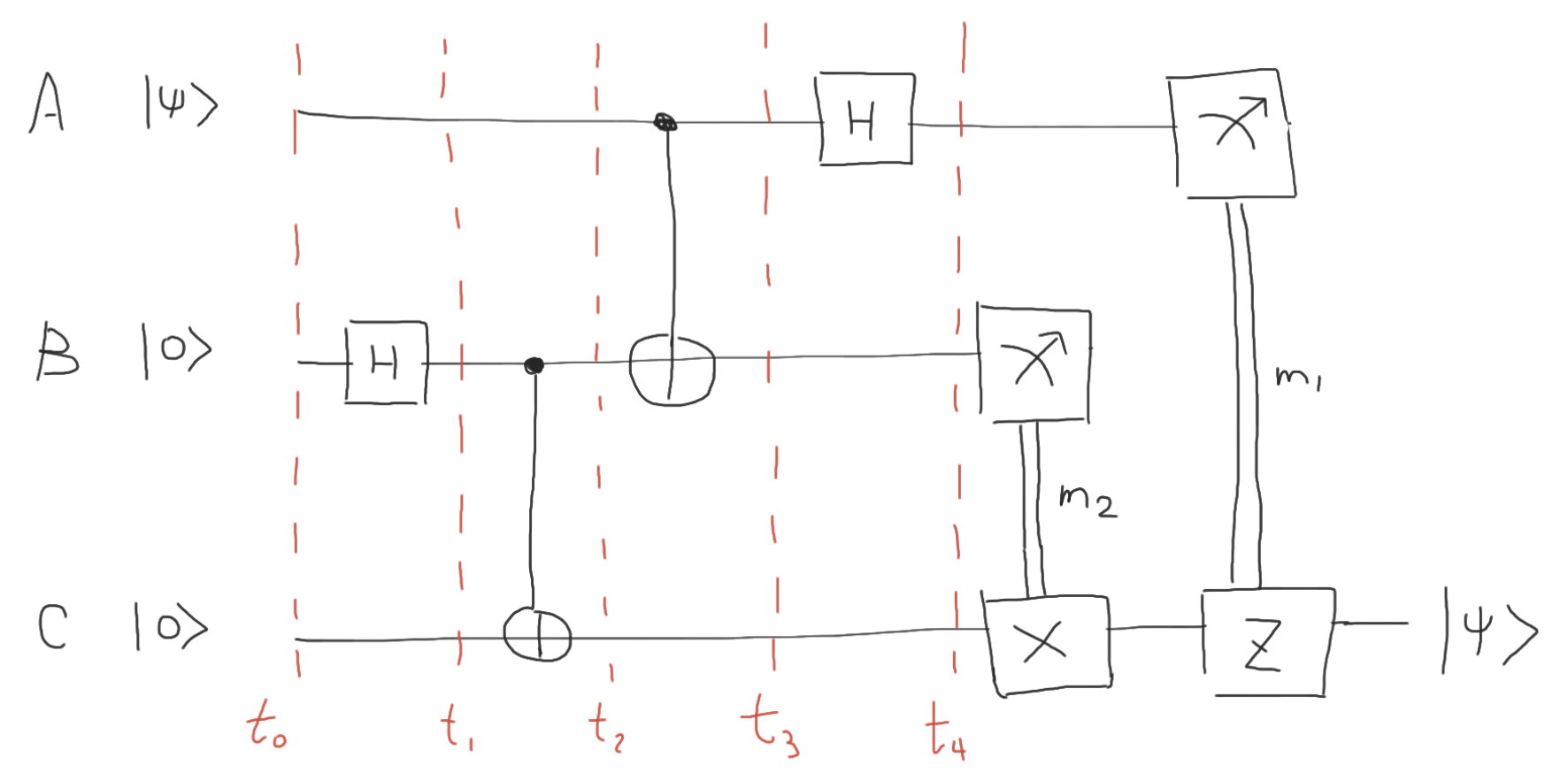### 起始狀態 ($$t_{0}$$):

A: $$\left| \psi \right> = \alpha \left| 0 \right> + \beta \left| 1 \right>$$

B: $$\left| 0 \right>$$

C: $$\left| 0 \right>$$

$\left| 0 \right> \otimes \left| 0 \right> = \left| 0 \right>\left| 0 \right> = \left| 00 \right>$

$t_{0}: (\alpha \left| 0 \right> + \beta \left| 1 \right>) \otimes \left| 0 \right> \otimes \left| 0 \right> = \alpha \left| 000 \right> + \beta \left| 100 \right>$

### 量子糾纏線路 ($$t_{0}$$ ~ $$t_{2}$$):

$$t_{0}$$ ~ $$t_{2}$$ 的這段線路主要是用來讓 B 和 C 進入量子糾纏狀態。經過任何量子閘時，我們要對所有的狀態進行計算。在進入 H Gate 之前，三個量子比特有兩個狀態，分別是 $$\alpha \left| 000 \right>$$ 和 $$\beta \left| 100 \right>$$ 。 由於只有 B 通過量子閘，轉換只會發生在第二個量子比特。透過之前的 H gate 真值表，我們可以算出在 $$t_{1}$$ 時的狀態。

$t_{1}: \alpha \left| 0 \right> H(\left| 0 \right>) \left| 0 \right> + \beta \left| 1 \right> H(\left| 0 \right>) \left| 0 \right>$ $t_{1}: \frac{\alpha}{\sqrt{2}} \left| 0 \right> (\left| 0 \right> + \left| 1 \right>) \left| 0 \right> + \frac{\beta}{\sqrt{2}} \left| 1 \right> (\left| 0 \right> + \left| 1 \right>) \left| 0 \right>$ $t_{1}: \frac{\alpha}{\sqrt{2}} \left| 000 \right> + \frac{\alpha}{\sqrt{2}} \left| 010 \right> + \frac{\beta}{\sqrt{2}} \left| 100 \right> + \frac{\beta}{\sqrt{2}} \left| 110 \right>$

$$t_{1}$$ 和 $$t_{2}$$ 之間有作用於 B 和 C 的 CNOT gate，控制點在 B。換句話說，只有當第二個量子比特的狀態是 $$\left| 1 \right>$$ 時，我們才需要反轉第三個量子比特。

$t_{2}: \frac{\alpha}{\sqrt{2}} \left| 000 \right> + \frac{\alpha}{\sqrt{2}} \left| 011 \right> + \frac{\beta}{\sqrt{2}} \left| 100 \right> + \frac{\beta}{\sqrt{2}} \left| 111 \right>$

### 出發地傳送線路 ($$t_{2}$$ ~ $$t_{4}$$):

$$t_{2}$$ 和 $$t_{3}$$ 之間也是 CNOT，只是換成了 A 和 B。

$t_{3}: \frac{\alpha}{\sqrt{2}} \left| 000 \right> + \frac{\alpha}{\sqrt{2}} \left| 011 \right> + \frac{\beta}{\sqrt{2}} \left| 110 \right> + \frac{\beta}{\sqrt{2}} \left| 101 \right>$

$$t_{4}$$ 則是 A 過了 H gate。值得注意的是 $$\frac{\alpha}{\sqrt{2}}$$ 變成了 $$\frac{\alpha}{2}$$，因為 H gate 會為等式帶來一個 $$\frac{1}{\sqrt{2}}$$。

$t_{4}: \frac{\alpha}{\sqrt{2}} H(\left| 0 \right>)\left| 00 \right> + \frac{\alpha}{\sqrt{2}} H(\left| 0 \right>)\left| 11 \right> + \frac{\beta}{\sqrt{2}} H(\left| 1 \right>)\left| 10 \right> + \frac{\beta}{\sqrt{2}} H(\left| 1 \right>) \left| 01 \right>$ $t_{4}: \frac{\alpha}{2} (\left| 0 \right> + \left| 1 \right>) \left| 00 \right> + \frac{\alpha}{2} (\left| 0 \right> + \left| 1 \right>) \left| 11 \right> + \frac{\beta}{2} (\left| 0 \right> - \left| 1 \right>) \left| 10 \right> + \frac{\beta}{2} (\left| 0 \right> - \left| 1 \right>) \left| 01 \right>$

$t_{4}: \frac{\alpha}{2} \left| 000 \right> + \frac{\alpha}{2} \left| 100 \right> + \frac{\alpha}{2} \left| 011 \right> + \frac{\alpha}{2} \left| 111 \right> + \frac{\beta}{2} \left| 010 \right> - \frac{\beta}{2} \left| 110 \right> + \frac{\beta}{2} \left| 001 \right> - \frac{\beta}{2} \left| 101 \right>$

$t_{4}: \frac{\alpha}{2} \left| 000 \right> + \frac{\beta}{2} \left| 001 \right> + \frac{\alpha}{2} \left| 011 \right> + \frac{\beta}{2} \left| 010 \right> + \frac{\alpha}{2} \left| 100 \right> - \frac{\beta}{2} \left| 101 \right> + \frac{\alpha}{2} \left| 111 \right> - \frac{\beta}{2} \left| 110 \right>$

$t_{4}: \frac{1}{2} \left| 00 \right> (\alpha \left| 0 \right> + \beta \left| 1 \right>) + \frac{1}{2} \left| 01 \right> (\alpha \left| 1 \right> + \beta \left| 0 \right>) + \frac{1}{2} \left| 10 \right> (\alpha \left| 0 \right> - \beta \left| 1 \right>) + \frac{1}{2} \left| 11 \right> (\alpha \left| 1 \right> - \beta \left| 0 \right>)$

### 出發地傳送線路 ($$t_{4}$$ 之後):#### A = 0, B = 0

C 的狀態： $$\alpha \left| 0 \right> + \beta \left| 1 \right>$$

#### A = 0, B = 1

C 的狀態： $$\alpha \left| 1 \right> + \beta \left| 0 \right>$$

$X(\alpha \left| 1 \right> + \beta \left| 0 \right>) = \alpha X(\left| 1 \right>) + \beta X(\left| 0 \right>) = \alpha \left| 0 \right> + \beta \left| 1 \right>$

#### A = 1, B = 0

C 的狀態： $$\alpha \left| 0 \right> - \beta \left| 1 \right>$$

$Z(\alpha \left| 0 \right> - \beta \left| 1 \right>) = \alpha Z(\left| 0 \right>) - \beta Z(\left| 1 \right>) = \alpha \left| 0 \right> + \beta \left| 1 \right>$

#### A = 1, B = 1

C 的狀態： $$\alpha \left| 1 \right> - \beta \left| 0 \right>$$

$X(\alpha \left| 1 \right> - \beta \left| 0 \right>) = \alpha X(\left| 1 \right>) - \beta X(\left| 0 \right>) = \alpha \left| 0 \right> - \beta \left| 1 \right>$

## 現實中的實現Hello, I'm Rhadow. A software engineer curious about how nature works. Dreaming to simulate our world in computer one day.